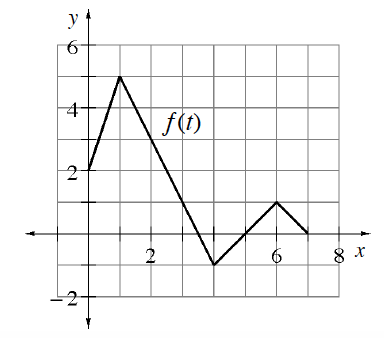### Home > APCALC > Chapter 9 > Lesson 9.1.2 > Problem9-28

9-28.

At right is a graph of $y = f(t)$. If $f(x) =\int _ { 1 } ^ { x } f ( t ) d t$ then determine:

1. $F(0)$

$F(0)=\int_1^0f(t)dt$

The integral represents the area under the curve. Notice that the bounds are reversed.

1. $F(1)$

$F(1)=\int_1^1f(t)dt$

What is the value of an integral if the bounds are equal?

1. $F^\prime(3)$

$F^\prime(3)=\frac{d}{dx}\int_1^3f(t)dt$

If you evaluate the integral above, will your answer be a number or an expression?
What is the derivative of the number or expression?

1. $F(6)$

What is the area under the curve from $x = 1$ to $x = 6$?